Скачать презентацию Question What is worse for consumers than

• Количество слайдов: 9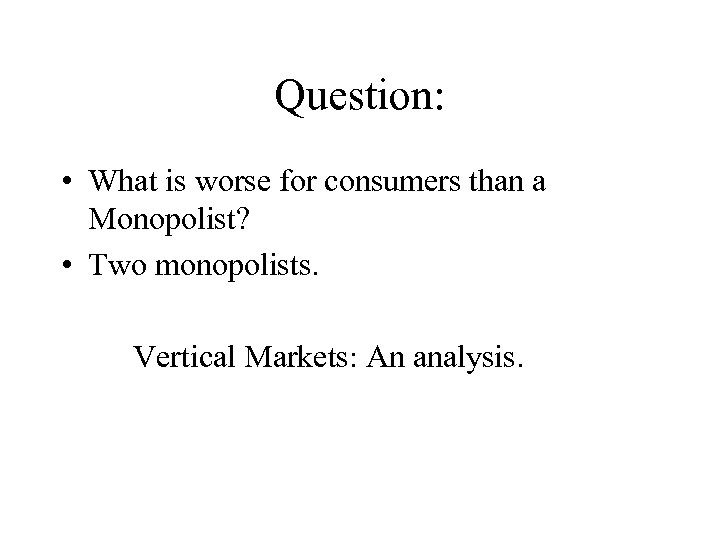Question: • What is worse for consumers than a Monopolist? • Two monopolists. Vertical Markets: An analysis.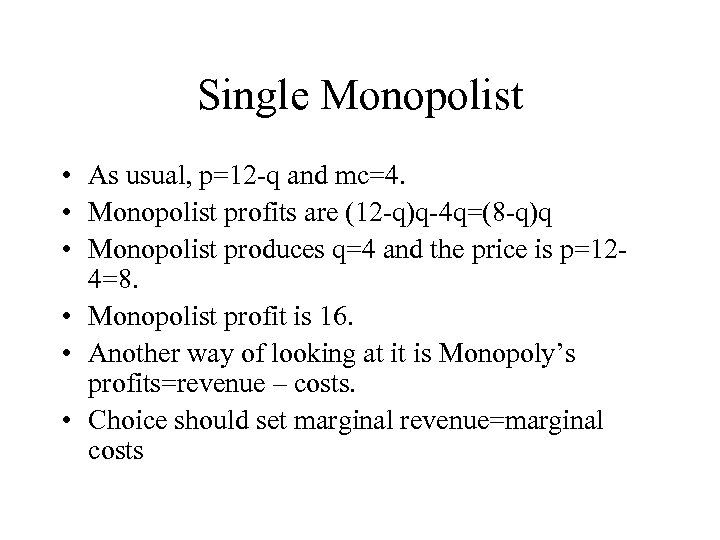Single Monopolist • As usual, p=12 -q and mc=4. • Monopolist profits are (12 -q)q-4 q=(8 -q)q • Monopolist produces q=4 and the price is p=124=8. • Monopolist profit is 16. • Another way of looking at it is Monopoly’s profits=revenue – costs. • Choice should set marginal revenue=marginal costs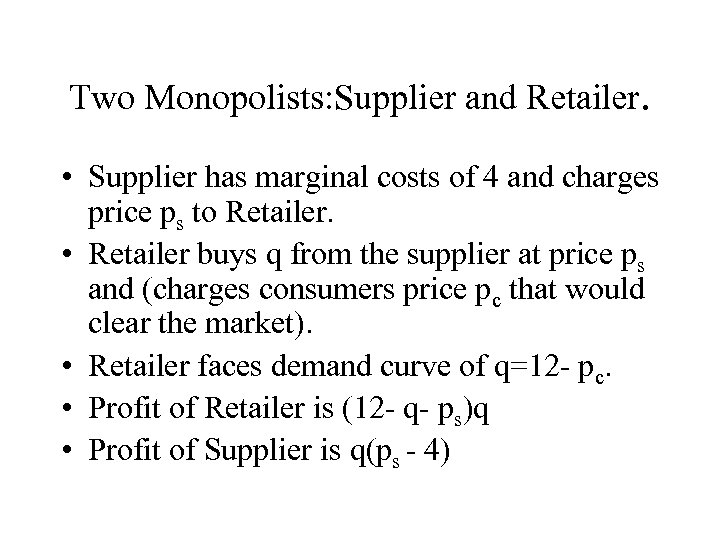Two Monopolists: Supplier and Retailer. • Supplier has marginal costs of 4 and charges price ps to Retailer. • Retailer buys q from the supplier at price ps and (charges consumers price pc that would clear the market). • Retailer faces demand curve of q=12 - pc. • Profit of Retailer is (12 - q- ps)q • Profit of Supplier is q(ps - 4)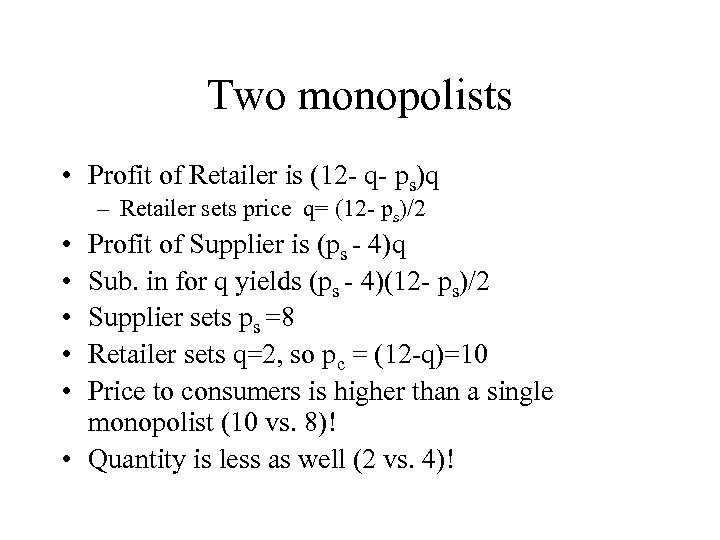Two monopolists • Profit of Retailer is (12 - q- ps)q – Retailer sets price q= (12 - ps)/2 • • • Profit of Supplier is (ps - 4)q Sub. in for q yields (ps - 4)(12 - ps)/2 Supplier sets ps =8 Retailer sets q=2, so pc = (12 -q)=10 Price to consumers is higher than a single monopolist (10 vs. 8)! • Quantity is less as well (2 vs. 4)!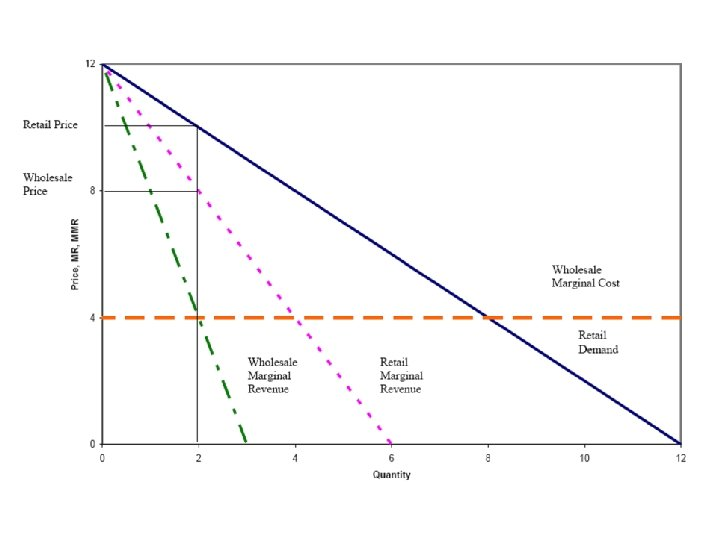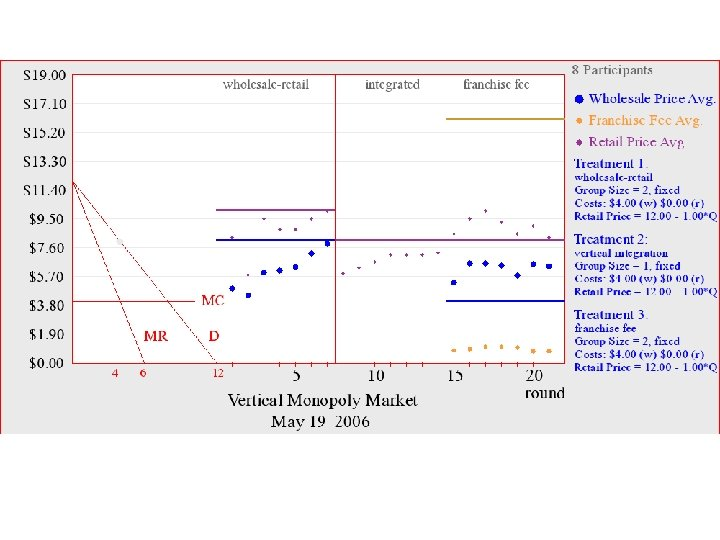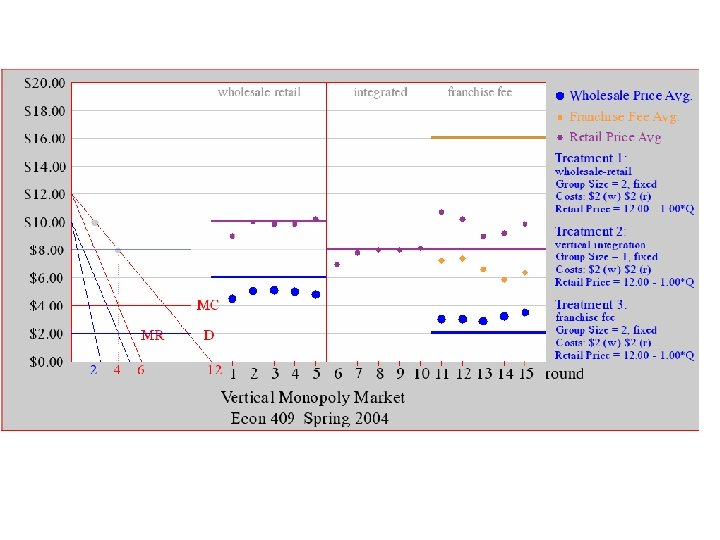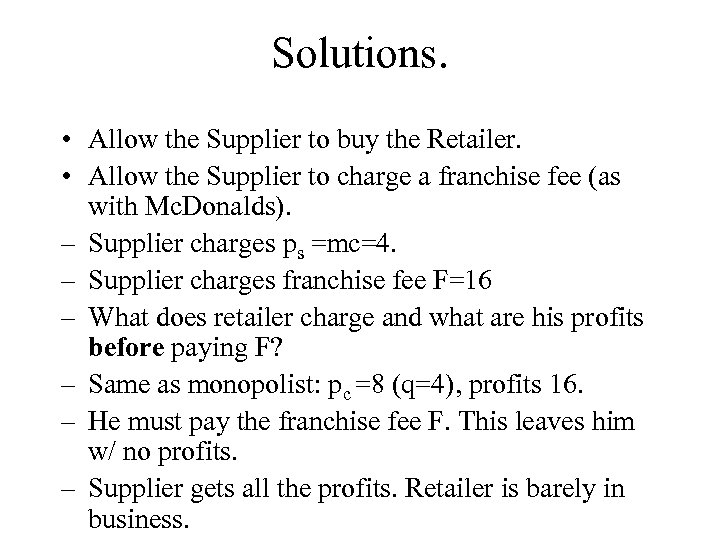Solutions. • Allow the Supplier to buy the Retailer. • Allow the Supplier to charge a franchise fee (as with Mc. Donalds). – Supplier charges ps =mc=4. – Supplier charges franchise fee F=16 – What does retailer charge and what are his profits before paying F? – Same as monopolist: pc =8 (q=4), profits 16. – He must pay the franchise fee F. This leaves him w/ no profits. – Supplier gets all the profits. Retailer is barely in business.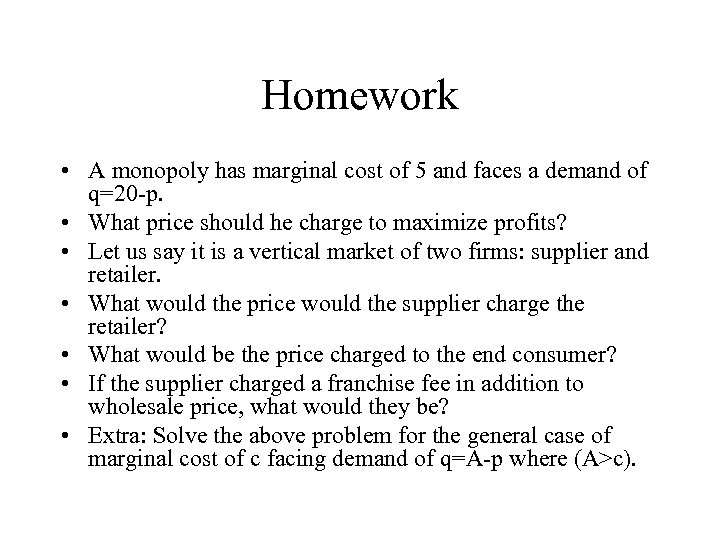Homework • A monopoly has marginal cost of 5 and faces a demand of q=20 -p. • What price should he charge to maximize profits? • Let us say it is a vertical market of two firms: supplier and retailer. • What would the price would the supplier charge the retailer? • What would be the price charged to the end consumer? • If the supplier charged a franchise fee in addition to wholesale price, what would they be? • Extra: Solve the above problem for the general case of marginal cost of c facing demand of q=A-p where (A>c).